# Symbols in Algebra

## Common Symbols Used in Algebra

Symbols save time and space when writing. Here are the most common algebraic symbols:

Symbol Meaning Example
+ add 3+7 = 10
subtract 5−2 = 3
× multiply 4×3 = 12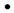multiply (as "×" can look like "x") 4·3 = 12
/ divide 20/5 = 4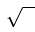square root ("radical") √4 = 2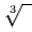cube root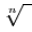nth root
( ) grouping symbols (round brackets) 2(a−3)
[ ] grouping symbols (square brackets) 2[ a−3(b+c) ]
{ } set symbols (curly brackets) {1,2,3}
= equals 1+1 = 2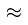approximately equal to π3.14
not equal to π 2
< ≤ less than, less than or equal to 2 < 3
> ≥ greater than, greater than or equal to 5 > 1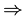implies (if ... then) a and b are odda+b is even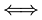"if and only if" or iff or "is equivalent to" x=y+1y=x−1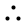Therefore a=bb=a
! Factorial 4! = 4×3×2×1 = 24